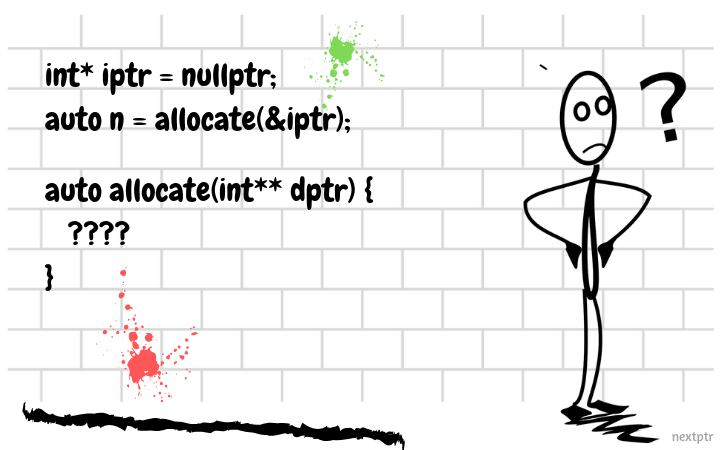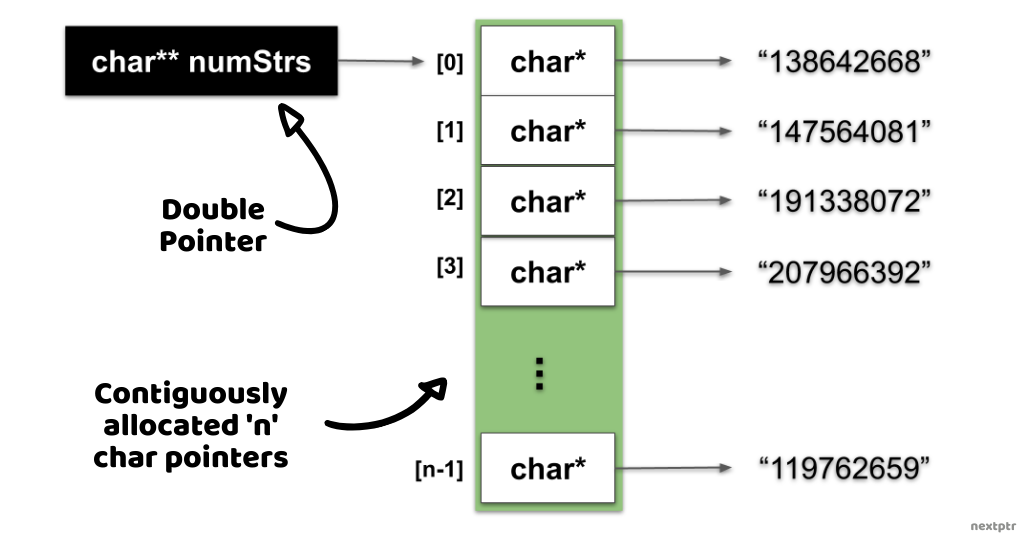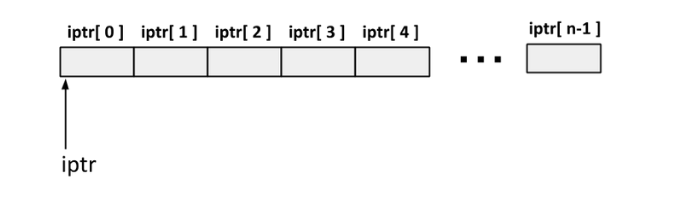# Using a double pointer for dynamic memory allocation## What is a double-pointer?

A double-pointer is a pointer to a pointer. For instance, a `char` pointer to pointer is declared as `char** ptrptr`.

Let's look at a real-life example of using a `char` double-pointer. In the following code, the function genNumStrings() returns a dynamic array of variable length number strings ("12345", "2345666", "12133131", ....). The genNumStrings() generates a given 'n' number of strings using the random number generator and fills them in a dynamic array of `char` pointers (`char*`). The dynamic array of `char*` itself is nothing but a double-pointer `char**`, as shown below:

``````//Returns a list of 'n' random number strings.
//Each number string is of a random length.
char** genNumStrings(size_t n) {
//Create a dynamic array of char*
//numStrs is a double-pointer
char** numStrs = new char*[n];

//seed the random num generator with current time.
std::srand((uint32_t)std::time(nullptr));

for(size_t i=0; i < n; i++) {
//Create a random number string
char str;
std::snprintf(str, sizeof str, "%d", std::rand());

//Allocate memory for one number string
//Store the char* pointer in the numStrs array
numStrs[i] = new char[std::strlen(str)+1];

//Copy the number string to the dynamic location
std::strcpy(numStrs[i], str); //safe
}
//Return char** double-pointer
return numStrs;
}
``````

The following illustration shows the memory layout of the dynamic array returned by the genNumStrings():The memory allocated by genNumStrings() can be freed by the function freeNumStrings(), as shown below:

``````//Frees the memory of all strings (char*)
//and the char**.
void freeNumStrings(char** numStrs, size_t n) {
//First free all the char*
for(int i=0; i < n; i++)
delete [] numStrs[i];
//Now free the char** itself
delete [] numStrs;
}
``````

Both of the above functions can be used as:

``````int main() {
auto numStrs = genNumStrings(10);
//Print all strings
for(int i=0; i < 10; i++)
std::cout << numStrs[i] << "\n";
//Free
freeNumStrings(numStrs, 10);
return 0;
}
``````

Having looked at the basics of the double-pointer, let's look at another example and a question in the next section.

## A Question

In the following code, an `int` pointer is dynamically assigned memory for a variable number of `int`s through a function allocate:

``````int* iptr = nullptr;
// allocate memory
auto n = allocate(&iptr);
``````

Once allocate returns, the iptr points to a memory location that holds n number of ints as shown:Below is the partial implementation of function allocate. It accepts an `int**` as an argument and returns the number or count of ints for which the memory is allocated:

``````auto allocate(int** dptr) {
size_t count = getCount();
/*__Allocate Memory__*/
return count;
}
``````

The function getCount above, which is not shown here, could be a function that reads from a database and returns the count of ints for which the memory is to be allocated. You have to select one from below choices that correctly allocates memory (or replaces `/*__Allocate Memory__*/`) in function allocate above (check `Explanations` for details):